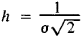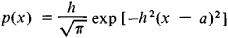# precision

(redirected from imprecision)
Also found in: Dictionary, Thesaurus, Medical, Legal, Wikipedia.

## precision

[prə′sizh·ən]
(mathematics)
The number of digits in a decimal fraction to the right of the decimal point.
(navigation)
In air operations, pertaining to a navigational facility which provides a combined azimuth and glide slope guidance to a runway.
(science and technology)
The measure of the range of values of a set of measurements; indicates reproducibility of the observations.

## Precision

a measure of the dispersion of the values of a random variable. The precision h is related to the standard deviation σ by the formulaThis method of measuring dispersion is justified since, in the case of a normal distribution, the probability density of a random variable with precision h and mathematical expectation a is described by the formulaThe precision is used as a measure of the dispersion primarily in gunnery theory and the theory of errors.

## precision

(mathematics)
The number of decimal places to which a number is computed.

Compare accuracy.

## precision

The number of digits used to express the fractional part of a number. The more digits, the more precision. See single precision and double precision. See also accuracy.
References in periodicals archive ?
A statistical approach was used to estimate the relative combined uncertainty (UC) by combining relative uncertainties of imprecision (Uimp), bias (Ub) and calibrators (Uref).
Error-transformed values at the extreme limits for the allowable errors for bias and imprecision for nonHDL demonstrate a large constant bias and a small proportional bias in relationship with the error-free nonHDL values (Figure 1).
Thus, 100 patients were modeled for 100 h with each regimen with both the hourly and every-5-min measurement schemas under 45 combinations of simulated bias and imprecision.
showed (3), the maximum allowable bias and imprecision are interrelated, and they can be described in the curve presented in Fig.
The imprecision data from QC material obtained over the duration of the trial is summarized in Table 1, together with the imprecision data obtained from duplicate testing on patient samples in the closest corresponding bin.
Data are presented as contour plots, which show the percent of insulin dosing errors as a function of increasing bias and imprecision.
A similar study that used different modeling techniques explored the effect of assay imprecision on the ROC area under the curve for prognosis by using cardiac troponin from the Gusto IIA trial (8).
1 is that total imprecision and random patient interferences have been separated.
The within-run imprecision (CV) and cardiac troponin I (cTnI) concentration pairs we derived from that study produce an SD profile that decreases slightly for cTnI concentrations of 0.
To assess the effect of increased assay imprecision on the estimate of the AUC and the SE of the AUC, we assumed that replicate measurements by a given assay of a sample with a fixed concentration of analyte followed a gaussian distribution and that the increase in assay CV was a constant percentage value across all concentrations within the measuring range of the assay.
1 are the probabilities of at least 1 of 3 serially measured values exceeding the 99th percentile limits as a function of the cTnI concentration for the 2 different imprecision profiles.
Imprecision was assessed in laboratories 1, 2, and 3 by use of commercial control samples, all prepared from human serum, obtained from three suppliers: Bio-Rad Laboratories BV; UTAK Laboratories Inc.

Site: Follow: Share:
Open / Close